# Difference between revisions of "Sine-cubed function"

This article is about a particular function from a subset of the real numbers to the real numbers. Information about the function, including its domain, range, and key data relating to graphing, differentiation, and integration, is presented in the article.
View a complete list of particular functions on this wiki
For functions involving angles (trigonometric functions, inverse trigonometric functions, etc.) we follow the convention that all angles are measured in radians. Thus, for instance, the angle of$90\,^\circ$ is measured as$\pi/2$.

## Definition

This function, denoted$\sin^3$, is defined as the composite of the cube function and the sine function. Explicitly, it is the map: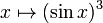$\! x \mapsto (\sin x)^3$

For brevity, we write$(\sin x)^3$ or$\sin^3x$.

## Key data

Item Value
Default domain all real numbers, i.e., all of$\R$
range the closed interval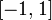$[-1,1]$, i.e.,$\{ y \mid -1 \le y \le 1 \}$
absolute maximum value: 1, absolute minimum value: -1
period$2\pi$, i.e.,$360\,^\circ$
local maximum values and points of attainment All local maximum values are equal to 1, and they are attained at all points of the form$2n\pi + \pi/2$ where$n$ varies over integers.
local minimum values and points of attainment All local minimum values are equal to -1, and they are attained at all points of the form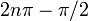$2n\pi - \pi/2$ where$n$ varies over integers.
points of inflection (both coordinates) All points of the form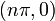$(n\pi,0)$, as well as points of the form$(n\pi + \alpha, (-1)^n2\sqrt{2}/3\sqrt{3})$ and$(n\pi + \pi - \alpha, (-1)^n2\sqrt{2}/3\sqrt{3})$ where$\alpha = \arcsin(\sqrt{2/3})$ where$n$ varies over integers.
derivative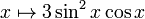$x \mapsto 3\sin^2x \cos x$
second derivative$x \mapsto 6 \sin x \cos^2x - 3\sin^3x = 6 \sin x - 9 \sin^3x$
antiderivative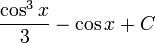$\frac{\cos^3x}{3} - \cos x + C$